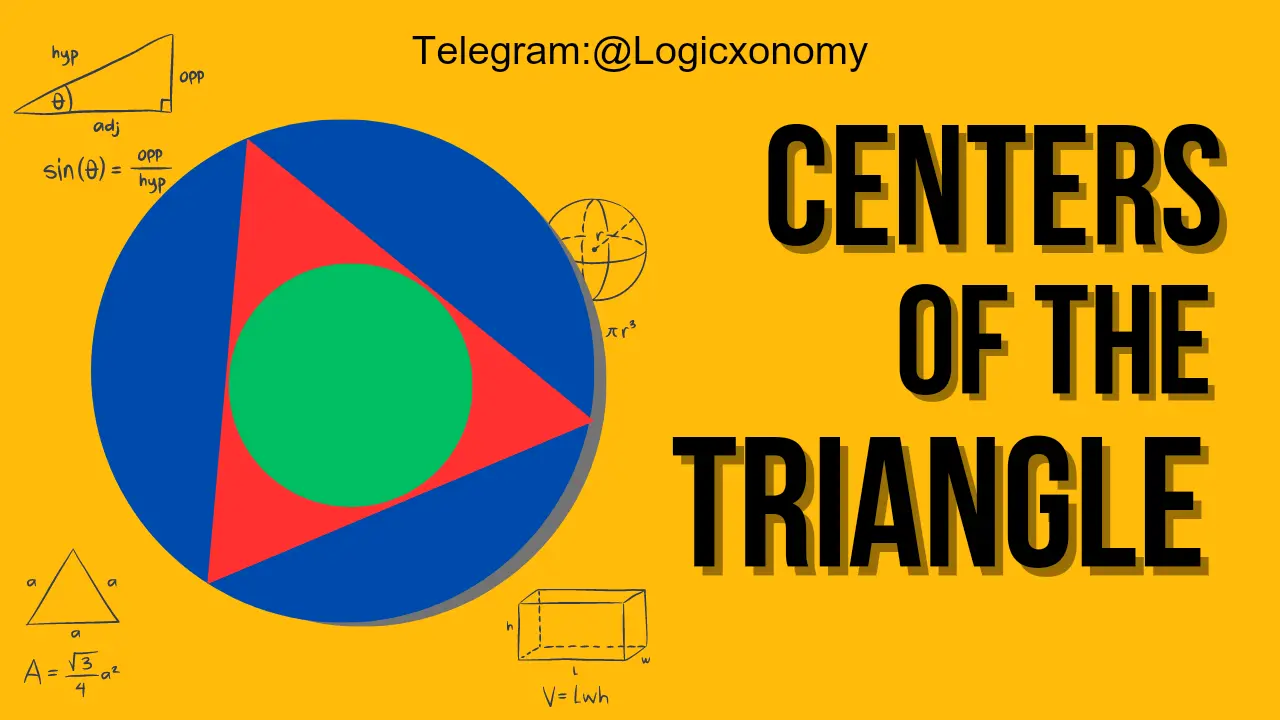Contents

The centers of a triangle – Incenter, Circumcenter, Centroid, Orthocenter, and Excenter – are fundamental to the study of geometry. Each of these centers has a unique location and set of properties that help define the triangle. In this chapter, we will study each of them one by one.

## Incenter

The point of intersection of two or more angle bisectors of a triangle is called the incentre of the triangle. It is denoted by ‘I’, where I is equidistant from all the sides of the triangle. It is the center of the circle that is inscribed inside the triangle and touches all three sides of the triangle.

In this given figure: ID=IE=IF=r

Here ‘r’ is the Inradius of the triangle

△=r×s ………(i)

‘s’ is the Semi-Perimeter of the triangle

△ is the Area of the Triangle

### Angle Bisector Theorem

The angle bisector drawn from any vertex of a triangle to the opposite side divides that side into segments that are proportional to the adjacent sides of the vertex.

BD: DC=c:b

AE: EC=c:a

BF: FA=a:b

AI: ID=b+c: a

BI: IE=a+c: b

CI: IF=a+b: c

### Angle made at the Incenter

∠BIC=90°+A/2

∠CIA=90°+B/2

∠AIB=90°+C/2

It means angles made at the incenter are Obtuse Angles.

### Inradius of the Right Angle Triangle

In △ABC, B=90° and O is the incenter. So altitudes OD=OE=OF=r

AB=p

BC=b

AC=hCenters of a Triangle$r=\frac{p+b-h}{2}$

### Coordinates of the Incenter

Let the coordinates of the vertices are A(x1,y1), B(x2,y2), C(x3,y3)

The coordinates of I= $\frac{a.x_1+b.x_2+c.x_3}{a+b+c},\frac{a.y_1+b.y_2+c.y_3}{a+b+c}$.

### Distance Between Incenter and Vertex

In the above figure, R is the circumradius of the triangle.

$R=\frac{abc}{4\triangle}$.

AI×BI×CI=4Rr2

Some important results:

r=4R.Sin(A/2).Sin(B/2).Sin(C/2)

s=4R.Cos(A/2).Cos(B/2).Cos(C/2)

The length of the inradius of a triangle is always less than or equal to half the length of the smallest side.

### Important Questions

Que 1: In △ABC, sides AB=4 cm, BC=6 cm, CA=3 cm then find the length of Angle bisector AD. Here D is the point on side BC.